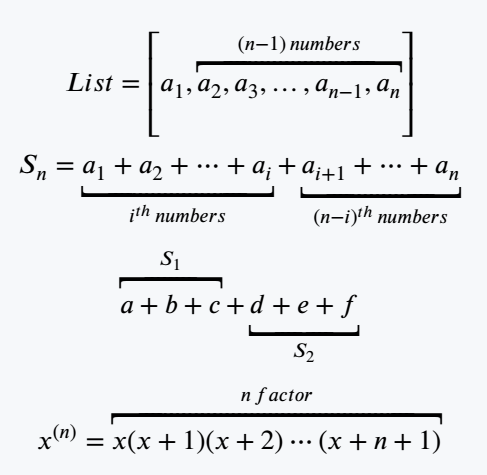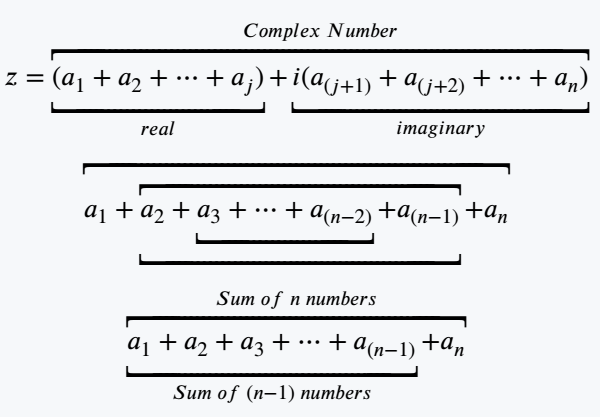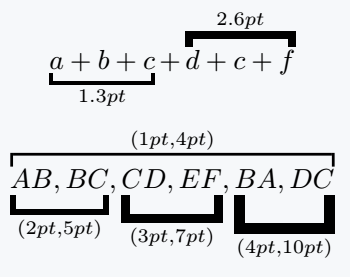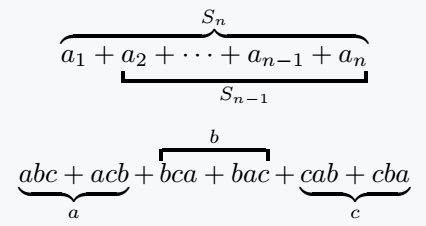# How to represent underbracket and overbracket in LaTeX?

You will face various problems with overbracket and underbracket. Because there is no default command in LaTeX to represent both expressions.

LaTeX has two commands, \underbracket and \overbracket, for the solution of this problem.

And by looking at the usage of both packages you can understand which is the best practice for representing both expressions.

## Use stix package for underbracket and overbracket

Same commands as overbrace and underbrace are defined in the stix package. Just bracket instead of a brace.

\documentclass{article}
\usepackage{stix}
\begin{document}
$List = \left[a_1,\overbracket{a_2,a_3,\dots,a_{n-1} ,a_n}^{(n-1)\,numbers}\right]$
$S_n = \underbracket{a_1+a_2+\cdots+a_i}_{i^{th}\,numbers}+\underbracket{a_{i+1}+\cdots+a_n}_{(n-i)^{th}\,numbers}$
$\overbracket{a+b+c}^{S_1}+\underbracket{d+e+f}_{S_2}$
$x^{(n)}=\overbracket{x(x+1)(x+2)\cdots (x+n+1)}^{n\,factor}$
\end{document}

Output :An expression is often observed where multiple underbrackets or overbrackets are vertically consecutive. In this case, the command must be passed between the command arguments to define the expression.

Of course, having a look at the latex code will make it easier to understand.

\documentclass{article}
\usepackage{stix}
\begin{document}
$z=\overbracket{\underbracket{(a_1+a_2+\cdots+a_j)}_{real}+\underbracket{i(a_{(j+1)}+a_{(j+2)}+\cdots+a_n)}_{imaginary}}^{Complex\;Number}$
$\overbracket{a_1+\overbracket{\underbracket{a_2+\underbracket{a_3+\cdots+a_{(n-2)}}+a_{(n-1)} }}+a_n}$
$\overbracket{\underbracket{a_1+a_2+a_3+\cdots+a_{(n-1)}}_{Sum\;of\; (n-1)\;numbers}+a_n}^{Sum\;of\;n\;numbers }$
\end{document}

Output :## Use mathtools package for extensible size of this symbol

Brackets are extensible in the mathtools package. So, you can change the thickness and height manually. As a result, you can give a more beautiful look to the expression.Same command exists within this package. But, if you want to change the thickness and height then you need to pass two optional arguments as values ​​with the command.

\documentclass{article}
\usepackage{mathtools}
\begin{document}
$\underbracket[1.3pt]{a+b+c}_{1.3pt}+\overbracket[2.6pt]{d+c+f}^{2.6pt}$
$\overbracket[1pt][4pt]{\underbracket[2pt][5pt]{AB,BC}_{(2pt,5pt)},\underbracket[3pt][7pt]{CD,EF}_{(3pt,7pt)},\underbracket[4pt][10pt]{BA,DC}_{(4pt,10pt)}}^{(1pt,4pt)}$
\end{document}

Output :If you pass only one optional argument, the thickness of the symbol will change.

## Use a combination of braces and bracket

Not only are multiple brackets used simultaneously, but braces are also used in many cases. Combination of braces and brackets can be used in same process.

\documentclass{article}
\usepackage{mathtools}
\begin{document}
$\underbracket[1.3pt]{a+b+c}_{1.3pt}+\overbracket[2.6pt]{d+c+f}^{2.6pt}$
$\overbracket[1pt][4pt]{\underbracket[2pt][5pt]{AB,BC}_{(2pt,5pt)},\underbracket[3pt][7pt]{CD,EF}_{(3pt,7pt)},\underbracket[4pt][10pt]{BA,DC}_{(4pt,10pt)}}^{(1pt,4pt)}$
\end{document}

Output :Comment option has been opened for you so that you can express your opinion. You tell me which package will be the best practice for this symbol!#### Md Jidan Mondal

LaTeX expert with over 10 years of experience in document preparation and typesetting. Specializes in creating professional documents, reports, and presentations using LaTeX.